11 Oct

Ilkke asked me how to create a nice chiptune snare. This question escalated into a challenge to write a little tutorial about how to make less boring chiptune drums. I used Klystrack to experiment but the basic theory holds for most programs and sound chips. You can hear the results below.

### Hi-hat

We’ll start with the easiest drum. Generally, just a stable noise waveform with a sharpish attack and a longer decay is enough. If a filter is available, filtering out the low frequencies with a high or band-pass filter helps to make the other rhythm sounds stand out – you don’t want the hi-hat to drown out the snare. For a shaker type sound, make the attack phase longer.

### Kick drum

Kick drum is a quite simple drum as well, if you need a basic techno kick or a longer 808-style sine wave oomph. The theory behind a basic kick goes like this: start high, finish low. Make the amplitude and the frequency drop sharply. If you hear a sharp decay in the frequency, you will perceive it like something punching through the other sounds. See an example below, it’s a generic 909 bass drum.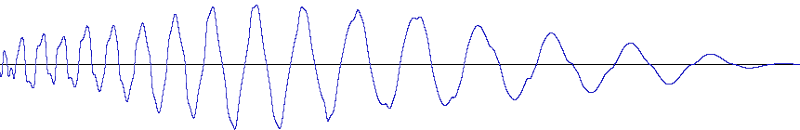However, if we look at a more interesting (and louder) kick sound, we will notice it’s not just a sine wave. There are higher frequencies there even though they are not that pronounced. A good thing to do is to combine the soft sine wave (triangle wave is a nice approximation) with something sharper and louder in the first moments of the kick. Try having a few milliseconds worth of square wave and/or noise in the beginning of the drum. Then continue as usual with a low-frequency tail.

To simulate a distorted drum, use the square waveform. As in the example below, you can see a hard-limited sine wave looks like square wave: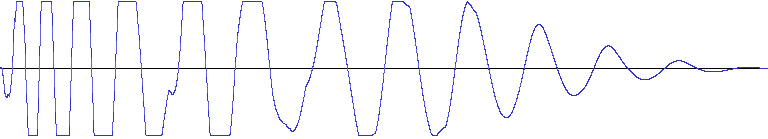An analysis of the kick drum sound used in Auf Wiedersehen Monty confirms the ideas discussed above: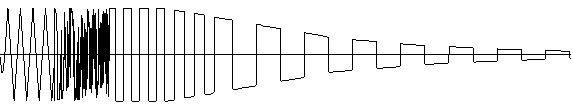[…]

1. it begins with a triangle wave, which is active for 20 mS [one program tick]

2. thereafter there is a short noise part for 20 mS

3. it ends with a pulse wave, the frequency and amplitude gets lower

### Snare drum

Creating a convincing snare drum starts to get a bit complicated. It helps if we once again look at what is actually happening in a good snare sound.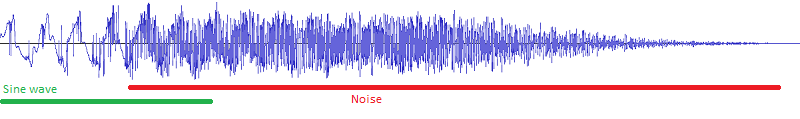As you can see, the snare sound consists of noise and a lower sine wave sound, the low frequency hum is the drum membrane vibrating. Also, the beginning of the hit the low sine wave is louder than the noisy rattle which adds a nice punch. We can emulate this by having a short pure triangle wave tone (use square wave to make it louder) in the beginning and change it to noise a moment later. As you can see, the waveform now looks a bit more like the original and has a beefy attack.

Another method to make an interesting snare often used by e.g. Rob Hubbard (I think) is to vary the noise frequency with a multi-octave arpeggio. If you need a real world analogue, you could think the cycling noise frequency (low-hi-low-hi-etc.) as reverberation.

### Closing thoughts and examples

So, what did we learn? Nature is interesting because it has tons of variation and is never static or perfect. For chiptune drums, this means: never use a single waveform or frequency.

1. Kick: Triangle wave only

2. Kick: Square wave

3. Kick: Square wave attack, triangle wave delay

4. Snare: Noise mixed with square and triangle, pure noise decay

5. Snare: Arpeggiated noise

02 Jan

## Introduction

Here’s an audio compression scheme I came up for my tracker project. Generally, I wanted an algorithm that meets these three conditions:

• Has to compress mono audio losslessly
• Has to be simple, should be just one source code file or 500 lines of C code at most
• Should compress 8-bit audio that’s upscaled to 16-bits to near 8 bits per sample

The lossless requirement is obvious and the simplicity gives the benefit of not having to rely on external libraries (e.g. when using something like RAR compression) and not being overkill. The audio data is not huge in any case so the compression ratio doesn’t have to be exceptional. The last requirement is the most important: in klystrack, it is possible to load raw 8-bit audio files, usually originally from the Amiga computer. The native audio format in klystrack is 16-bit, however, so when saving the audio data there always are 8 redundant bits and the file will generally be twice as large as the original file. Also, since the software deals with low-fidelity aesthetics (chiptunes), the audio data might be even lower quality than 8-bit.

While there’s the option of detecting the bit fidelity and only saving the most significant bits, I thought it should be quite simple to devise an algorithm that automatically detects and leaves the redundant bits out while still doing some compression for even 16-bit audio data.

## The algorithm

In short, the algorithm does this:

1. Delta code input data

2. Gray code the data from above

3. Reorder the data from above in bit planes. I.e. first output the least significant bit of all samples, then the next bit for all samples and so forth

4. Run-length encode the above data exploiting the fact you only need to store the distance between bit flips

### Delta encoding

By delta encoding the audio data, we can exploit the fact audio data very often changes just a little between samples and so the generated delta values (the amount of change) need fewer bits to be expressed. However, you can’t store 16-bit data with e.g. 8-bit delta values since it is not assured the change is always small enough to be stored in 8 bits, requiring the use of 16-bit delta values in the worst case.

### Gray encoding

Using gray codes we can minimize the amount of bit flipping that happens (e.g. when a value changes between 7 and 8, where the bits change from 0111 to 1000 — three bits flip even if the value changes by one) to one bit flip between any two neighboring values. In practice, this doesn’t always result in better compression and sometimes it even can hurt the compression ratio.

### Reordering data in bit planes

This step is the key to good compression in our case. Between two neighboring delta values, it’s common that most of the bits are the same and it’s common for one bit to stay completely static for the whole data. So, we take the bits from all samples and sort them so that all significant bits come before the lesser bits. The result is long runs of bits, usually in the most significant bits.

### Run-length encoding

Finally, we take the encoded and reordered data and store the bits using RLE. While RLE usually stores the repeated data and the number of repetitions, we can exploit the fact the data is always zero or one, always changing between runs — only the starting state of the bit needs to be stored. Elias gamma coding is used to store the run-lengths since in most cases the run length is in the range of a few bits (for completely empty bit planes we can use special flags that tell they’re all zero or one) and tend to fall in 1-4 bits. With gamma coding it’s possible to store the number 1 by using one bit, the number two takes three bits.

Also, for some bit planes a raw dump of bits with no compression should be used if compression would result in much longer data than the original. There are also two special codes for the bit planes, in cases where all bits are zero or one (common if the audio data is quiet, i.e. uses only a subrange of the 16-bit resolution) or if the data is offset by a constant.

Depending on implementation the total overhead is around two bits per bit plane (i.e. 32 bits or two bytes for 16-bit audio data), not including the header data describing the compressed data (data length, uncompressed length). Since there’s the possibility of a raw dump of bits, in the worst case (none of the bit planes compress at all), the data size grows only by two bytes. A completely quiet (all zero bits) data will take only two bytes for any length.

## Improvements

My implementation only compresses the whole audio data as one big chunk but this can be easily improved to compress in chunks. This should improve the compression when there are parts where the audio is quiet and most of the bits will be zero.

Stereo audio can be compressed by compressing two chunks of data, one for both channels. The other channel should be delta coded compared to the other channel since in stereo audio the two channels often heavily correlate.

Since the Gray coding may even hurt compression, there should be the option to disable the coding for chunks. This can be simply by brute force, comparing which combination of options produces the shortest compressed data.

## Compression results

The following contains example compression results. The Option column tells different configurations where the input data is delta coded and/or Gray coded. This shows how different preprocessing steps work better for different kinds of input data.

Legend: 0 = no coding before compression, 1 = delta coding, 2 = Gray coding, 3 = delta and Gray coding.

Options Ratio
Sine wave (loud)
0 73.1 %
1 29.6 %
2 67.9 %
3 22.6 %
White noise (loud)
0 100.0 %
1 100.0 %
2 99.8 %
3 100.0 %
Complex wave
0 75.3 %
1 38.2 %
2 69.6 %
3 30.0 %
Options Ratio
Sine wave and noise
0 84.7 %
1 100.0 %
2 80.9 %
3 80.9 %
White noise (quiet)
0 50.0 %
1 100.0 %
2 50.0 %
3 55.9 %
Complex wave (saturated)
0 38.9 %
1 21.9 %
2 37.2 %
3 17.7 %

## Source code

```#include <stdio.h>
#include <stdlib.h>
#include <string.h>
#include <math.h>

typedef unsigned char Uint8;
typedef short int Sint16;
typedef unsigned short int Uint16;
typedef int Sint32;
typedef unsigned int Uint32;

/* bitpack() bit plane codes */
enum
{
BITPACK_STATIC0,
BITPACK_STATIC1,
BITPACK_LITERAL,
BITPACK_RLE
};

/* bitstream writer helpers*/
typedef struct
{
Uint8 *buffer;
Uint32 byte, size_byte; 	// Read/write position counters (byte component)
Uint8 bit, size_bit;		// Read/write position counters (bit component)
} BitPtr;

#define BIT_BLOCK_SIZE 1024 // Bitstream buffer allocation increment size

void bit_init(BitPtr *p, Uint8 *buffer, Uint32 size_bytes, Uint8 size_bits)
{
memset(p, 0, sizeof(*p));
p->buffer = buffer;
p->size_byte = size_bytes;
p->size_bit = size_bits;
}

void bit_deinit(BitPtr *p)
{
free(p->buffer);
memset(p, 0, sizeof(*p));
}

void bit_rewind(BitPtr *p)
{
p->bit = p->byte = 0;
}

void bit_seek(BitPtr *p, Uint32 byte, Uint8 bit)
{
p->byte = byte;
p->bit = bit;
}

void bit_w(BitPtr *p, int bit)
{
if (p->bit == 0 && !(p->byte & (BIT_BLOCK_SIZE - 1)))
{
// If write position modulo BIT_BLOCK_SIZE is zero, allocate a larger buffer
p->buffer = realloc(p->buffer, p->byte + BIT_BLOCK_SIZE);
}

if (bit)
p->buffer[p->byte] |= 1 << p->bit;
else
p->buffer[p->byte] &= ~(1 << p->bit);

++p->bit;

if (p->bit == 8)
{
p->bit = 0;
++p->byte;
}

p->size_byte = p->byte;
p->size_bit = p->bit;
}

int bit_r(BitPtr *p)
{
if (p->size_byte <= p->byte && p->size_bit <= p->bit)
return -1;

int bit = (p->buffer[p->byte] & (1 << p->bit)) != 0;

++p->bit;

if (p->bit == 8)
{
p->bit = 0;
++p->byte;
}

return bit;
}

/* Write Elias gamma coded value (has to be nonzero) */
void bit_wgamma(BitPtr *p, Uint32 value)
{
int l = log2(value);

for (int a=0; a < l; a++)
bit_w(p, 0); //put 0s to indicate how many bits will follow

bit_w(p, 1);      //mark the end of the 0s

for (int a=l-1; a >= 0; a--) //Write the bits as plain binary
{
bit_w(p, value & 1 << a);
}

}

/* Read Elias gamma coded value, zero return value signals read error */
Uint32 bit_rgamma(BitPtr *p)
{
int numberBits = 0;
int bit;
while (!(bit = bit_r(p)))
numberBits++; //keep on reading until we fetch a one...

if (bit < 0) return 0;

Uint32 current = 0;
for (int a=numberBits-1; a >= 0; a--) //Read numberBits bits
{
if ((bit = bit_r(p)))
current |= 1 << a;

if (bit < 0) return 0;
}
current |= 1 << numberBits; //last bit isn't encoded!

return current;
}

Sint32 bit_rbits(BitPtr *p, Uint8 bits)
{
Sint32 rval = 0;

for (int i = 0 ; i < bits ; ++i)
{
int bit = bit_r(p);

if (bit < 0) return -1;

rval |= bit << i;
}

return rval;
}

/* Write bits bits of v */
void bit_wbits(BitPtr *p, Uint32 v, Uint8 bits)
{
for (int i = 0 ; i < bits ; ++i)
{
bit_w(p, v & (1 << i));
}
}

/* Gray and delta encoding helpers */
static inline Uint16 gray(Uint16 v)
{
return v ^ (v >> 1);
}

static inline Uint16 degray(Uint16 v)
{
v ^= (v>>8);
v ^= (v>>4);
v ^= (v>>2);
v ^= (v>>1);
return v;
}

static void delta_encode(Sint16 *buffer, const int n)
{
Sint32 delta = 0;
Sint32 original;
for (int i = 0; i < n; ++i)
{
original = buffer[i];
Sint32 x = original - delta;
buffer[i] = gray(x + 32768); // Gray code can not be negative
delta = original;
}
}

static void delta_decode(Sint16 *buffer, const int n)
{
Sint32 delta = 0;
for (int i = 0; i < n; ++i)
{
Sint32 v = degray(buffer[i]);
buffer[i] = (v - 32768) + delta;
delta = buffer[i];
}
}

/* Compress 16-bit signed data into bitstream, return compressed data size in packed_size (in bits) */
Uint8 * bitpack(const Sint16 *_buffer, const int n, Uint32 *packed_size)
{
BitPtr bp;
bit_init(&bp, NULL, 0, 0);

Sint16 *buffer = malloc(sizeof(Sint16) * n);
memcpy(buffer, _buffer, sizeof(Sint16) * n);

delta_encode(buffer, n);

for (int plane = 0 ; plane < sizeof(*buffer) * 8 ; ++plane)
{
const Sint16 mask = 1 << plane;
int bit = mask & *buffer;

int type = BITPACK_STATIC0 | (bit != 0);

for (int i = 0 ; i < n ; ++i)
if ((buffer[i] & mask) != bit)
{
// Was not all zeros or all ones, needs to compress
type = BITPACK_RLE;
break;
}

Uint32 p_byte = bp.byte;
Uint32 p_bit = bp.bit;

again:

bit_wbits(&bp, type, 2);

switch (type)
{
case BITPACK_LITERAL:
for (int i = 0 ; i < n ; ++i)
break;

case BITPACK_STATIC0:
case BITPACK_STATIC1:
// No data needed, bit plane is all zeros or all ones
break;

case BITPACK_RLE:
{
// Write starting bit state
bit_w(&bp, bit);

Uint32 ctr = 0;

for (int i = 0 ; i < n ; ++i)
{
if (((buffer[i] & mask) == bit))
++ctr;

if ((buffer[i] & mask) != bit)
{
bit_wgamma(&bp, ctr);

ctr = 1;

// Flip the bit (no neighboring bits are the same state)
}
}

if (ctr != 0) bit_wgamma(&bp, ctr);

if ((bp.byte * 8 + bp.bit) - (p_byte * 8 + p_bit) > n + 2)
{
// RLE gave longer data than the original, dump data instead
bit_seek(&bp, p_byte, p_bit);
type = BITPACK_LITERAL;
goto again;
}

}
break;
}

}

free(buffer);

*packed_size = bp.byte * 8 + bp.bit;

return bp.buffer;
}

/* Decompress compressed bitstream into 16-bit signed data, decompress at most packed_size bits
unpacked_size tells the function the data length (important)
*/
Sint16 * bitunpack(const Uint8 *packed_data, const Uint32 packed_size, Uint32 unpacked_size)
{
BitPtr bp;
bit_init(&bp, (Uint8*)packed_data, packed_size / 8, packed_size & 7);

Sint16 *buffer = calloc(unpacked_size, sizeof(Sint16));

for (int plane = 0 ; plane < sizeof(*buffer) * 8 ; ++plane)
{
const Sint16 mask = 1 << plane;

Sint32 type = bit_rbits(&bp, 2);

if (type < 0) goto read_error;

switch (type)
{
case BITPACK_LITERAL:
for (int i = 0 ; i < unpacked_size ; ++i)
{
int bit = bit_r(&bp);
if (bit < 0) goto read_error;
}
break;

case BITPACK_STATIC0:
// Data bits are zero by default, no action needed
break;

case BITPACK_STATIC1:
// Fill bit plane with set/unset bit
for (int i = 0 ; i < unpacked_size ; ++i)
break;

case BITPACK_RLE:
{
// Read the starting bit status
int bit = bit_r(&bp);

if (bit < 0) goto read_error;

if (bit) bit = mask; else bit = 0;

buffer |= bit;

for (int i = 0 ; i < unpacked_size ; )
{
Uint32 ctr = bit_rgamma(&bp);

if (ctr == 0) goto read_error;

for (; i < unpacked_size && ctr ; ++i, --ctr)
buffer[i] |= bit;

// Flip the bit (neighboring bits are always different)
}
}
break;
}
}

delta_decode(buffer, unpacked_size);

if (0)
{
free(buffer);
return NULL;
}

return buffer;
}

/* Generate test data, 0 is a sine wave and 1 generates noise */
void getdata(Sint16 *buffer, const int n, int t)
{
switch (t)
{
case 0:
for (int i = 0 ; i < n ; ++i)
{
buffer[i] = (((int)(sin((float)i / 1000 * 3.141 * 2) * 20000)));
}

break;

case 1:
for (int i = 0 ; i < n ; ++i)
buffer[i] = (rand() * 4);

break;
}
}

int main(int argc, char **argv)
{
const int n = 100000;

Sint16 *b = malloc(sizeof(Sint16) * n);
Uint32 packed_size;

getdata(b, n, 0);

Uint8 * data = bitpack(b, n, &packed_size);
Sint16 * udata = bitunpack(data, packed_size, n);

if (udata == NULL || memcmp(b, udata, sizeof(Sint16) * n) != 0)
{
printf("Upack failed\n");
}
else
{
printf("Compression ratio %.1f %%\n", (float)100 * packed_size / (n * sizeof(Sint16) * 8));

}

free(data);
free(udata);
free(b);

return 0;
}
```
10 Dec

Original song is “future” by buzzer, an AHX module imported in klystrack with no modifications.

29 Nov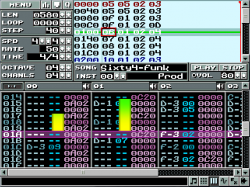After months of busy developing (not), I have finally decided to release klystrack 1.5. In short: feature creep. iLKke also joined the dev team and I have to thank him for graphics, testing and general support (motivational kicks in the butt). I also got some very good feedback from quite a few people, even though not every request was possible to implement (thanks nonetheless).

What is new in 1.5:

• Improved editing
• Undo/redo for most actions
• The “compact” pattern display mode can be configured to display exactly the needed pattern columns
• There’s now an edit mode similar to most classic trackers (you can jam in the pattern editor without changing pattern data)
• New audio driver features
• Wavetable for sampled drums etc.
• Bitcrush effect has now optional sample rate downsampling
• Extra parameter column for volume (like in FT2)
• New commands such as setting filter resonance and restarting the instrument program from pattern data
• Improved GUI
• Fun stuff
• VU meters and a fake spectrum analyzer (think Protracker)
• AHX/Protracker/C64 etc. retro themes
• Experimental stuff
• MIDI sync (not tested very well) and MIDI keyboard support (great for instrument editing)

Get it here. Don’t forget the Facebook page, updates get posted there more frequently.

16 Jun